### 文章目录

• 前言
• 一、傅里叶变换的离散性与周期性
• 二、MATLAB 实现快速傅里叶变换 FFT (DFT) 的计算
• 三，FFT 频谱的对称性
• 四，FFT 频谱的频率刻度
• 五，频谱图的绘制（半谱图&全谱图）
• 六 练习 绘制cos信号的频谱图
• 半谱图
• 全谱图
• 七，IFFT反傅里叶变换
• 八，采样规则
• 九，二维傅里叶变换

# 前言

◼ 傅里叶变换的好处
✓ 正弦信号比原信号更简单 → 已被充分地研究
✓ 处理正弦信号 → 比处理原信号更简单
✓ 线性系统 → 正弦信号的频率保持性
➢ 输入为正弦信号 → 输出仍是同频率的正弦信号
➢ 幅值和相位可能发生变化 → 但频率与输入信号保持一致
➢ 频率保持性具有很高的工程实用价值

# 一、傅里叶变换的离散性与周期性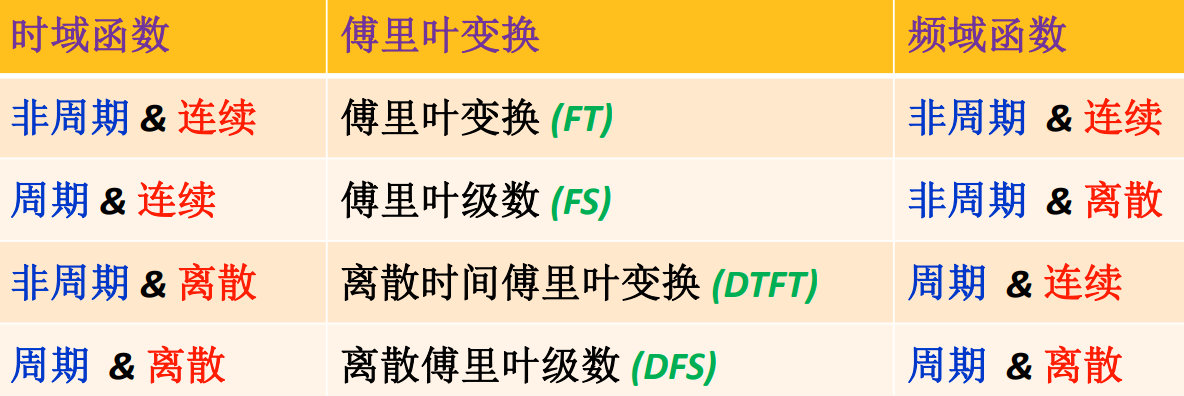FFT只是加速 DFT 运算的一种快速算法， 在信号理论上没有新的贡献 。其计算的结果和本质内容仍然是离散傅里叶变换 (DFT)

# 二、MATLAB 实现快速傅里叶变换 FFT (DFT) 的计算

MATLAB 函数的调用格式 → X = fft (x) // X = fft (x, n)
x 是一个时域序列 → 通常为时域信号的采样值
n 用于定义 → 进行 FFT 计算的数据个数
➢ 如果 n 大于 x 的长度 → 自动在 x 的末尾添加 0 → 使得 x 的长度等于 n
➢ 如果 n 小于 x 的长度 → 自动截取 x 中的前 n 个数来进行 FFT 计算
X 返回 FFT 的计算结果 → 通常是一个复数序列

# 三，FFT 频谱的对称性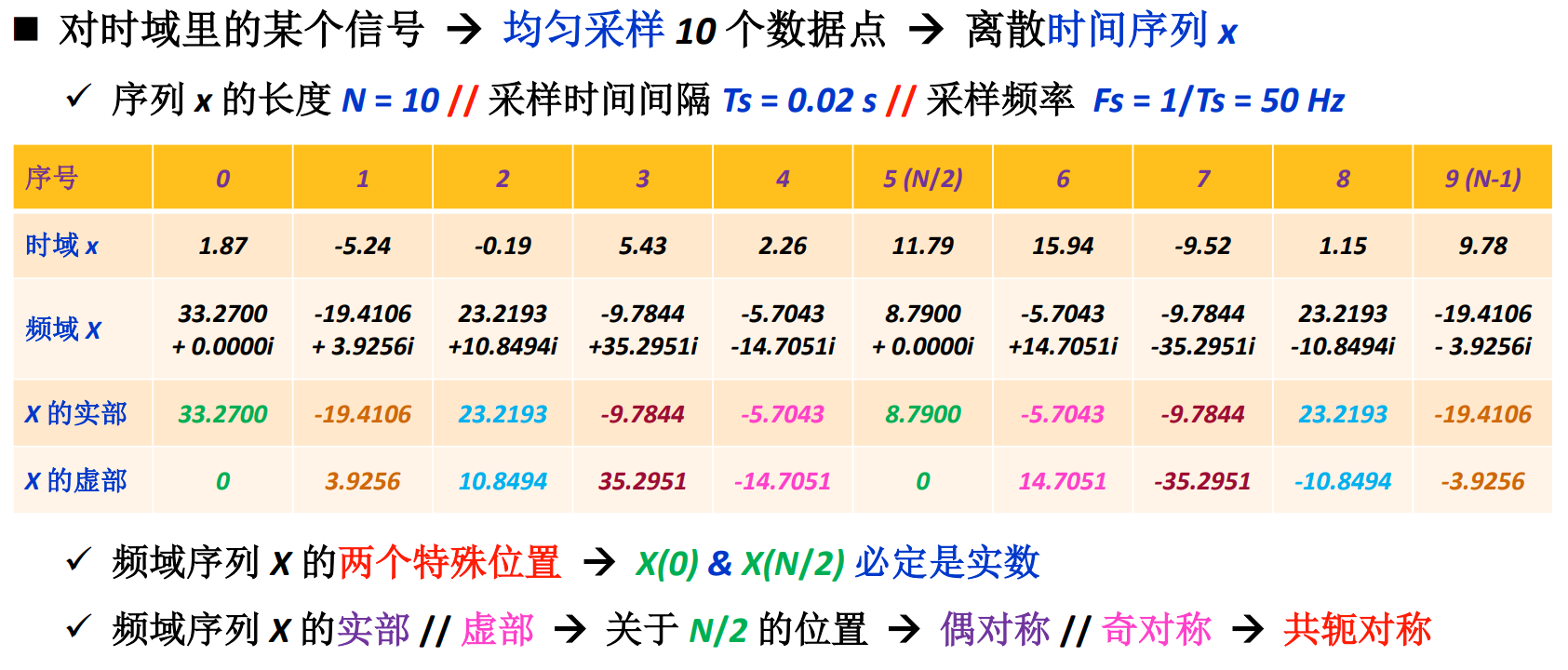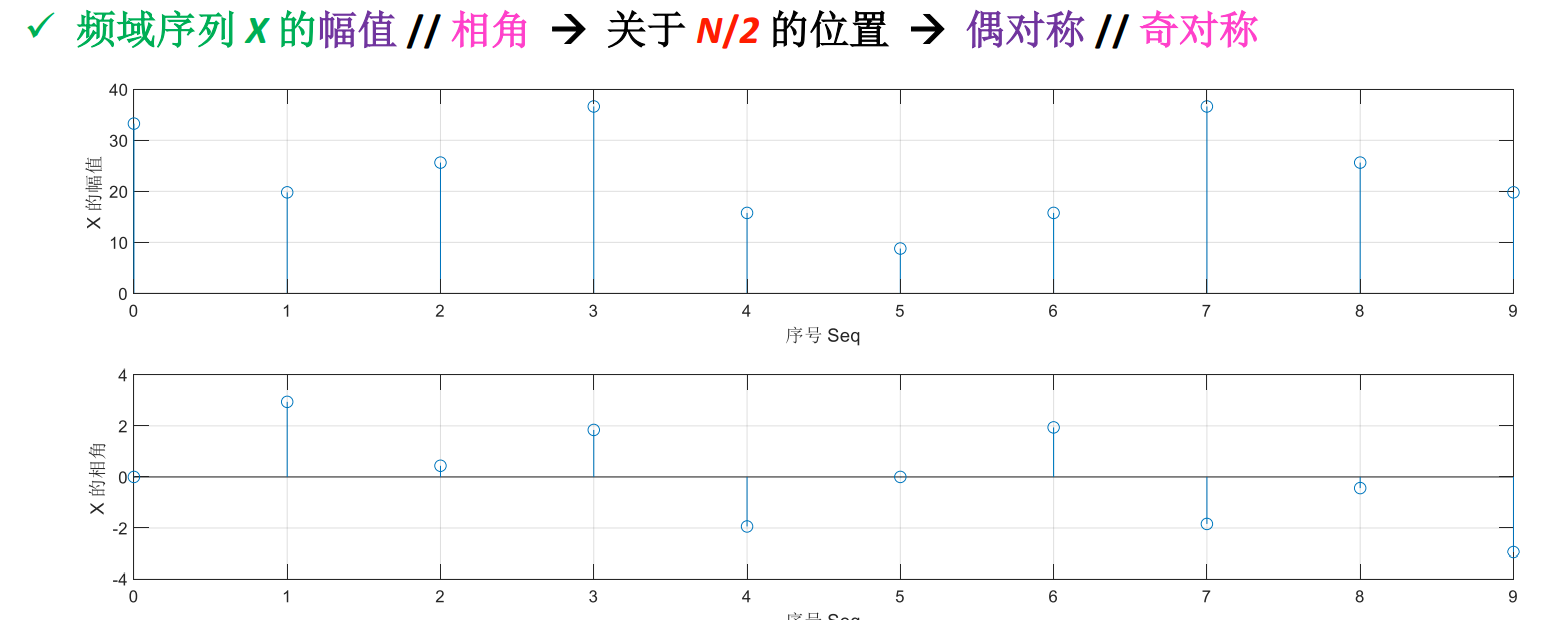# 四，FFT 频谱的频率刻度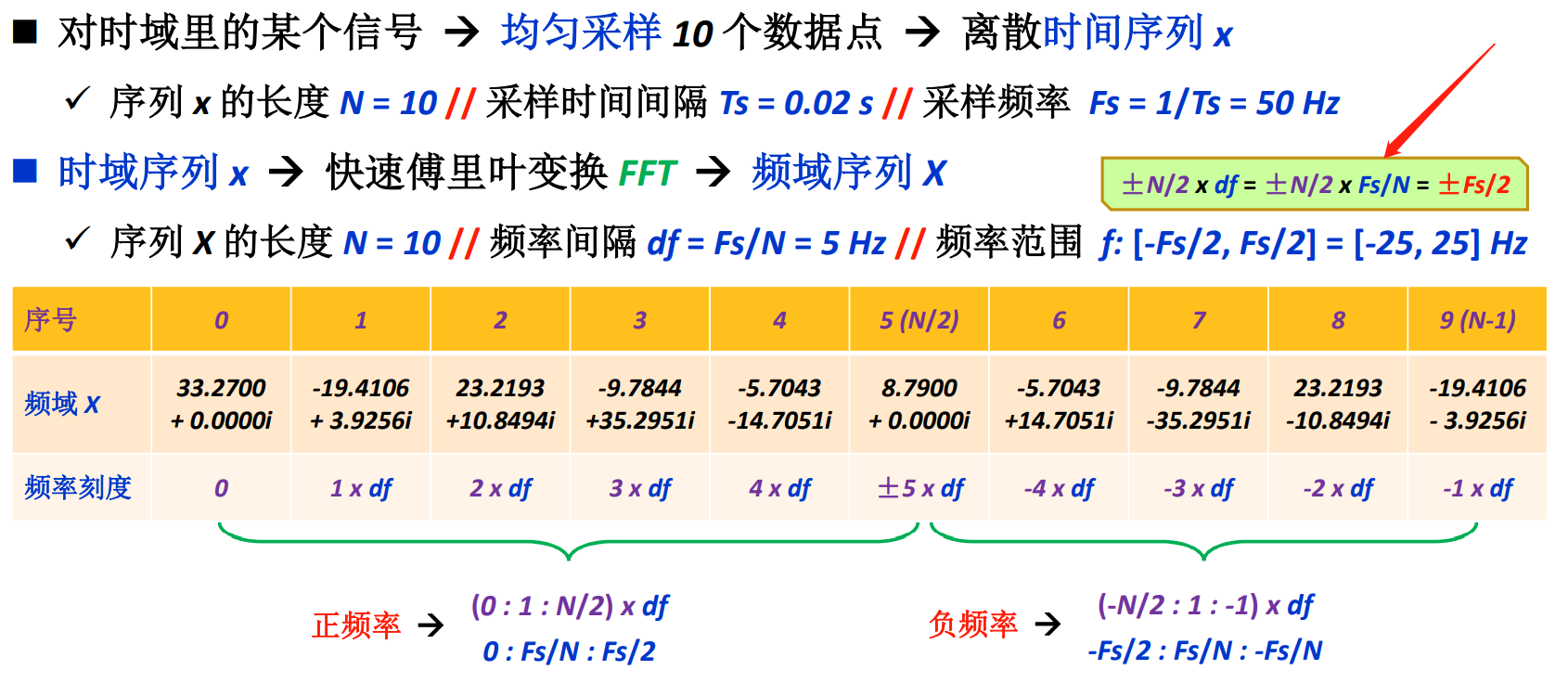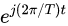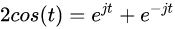# 五，频谱图的绘制（半谱图&全谱图）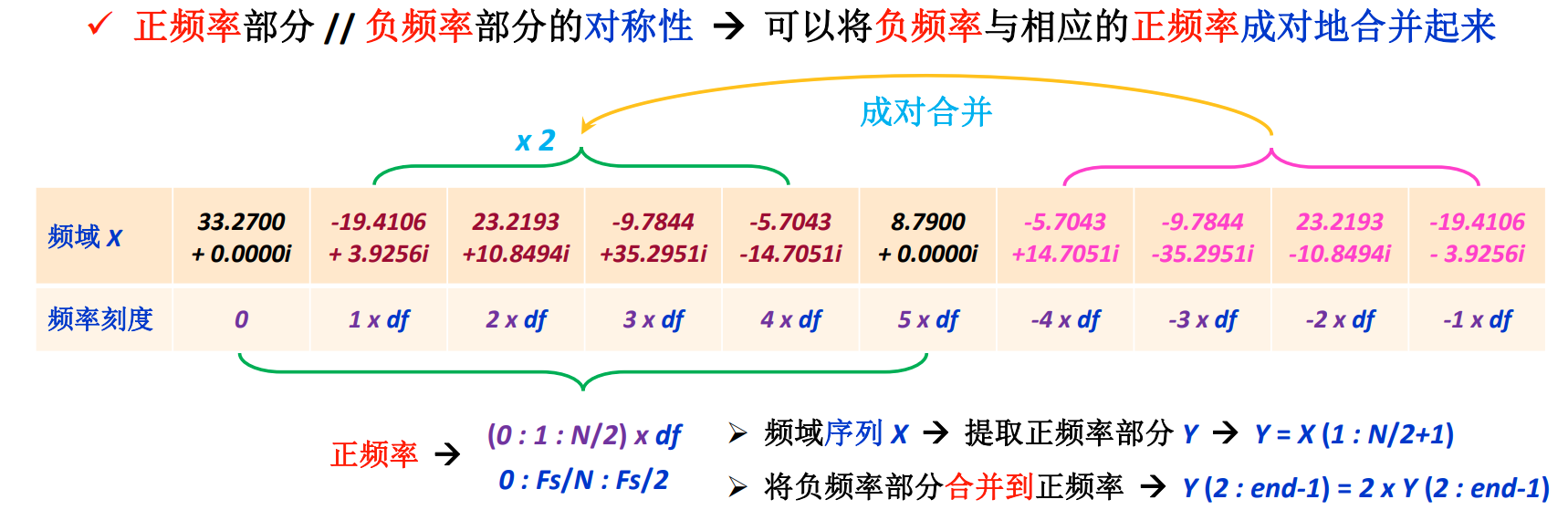序列 Y 对应的频率刻度 → (0 : 1 : N/2) x df
→ 0 : Fs/N : Fs/2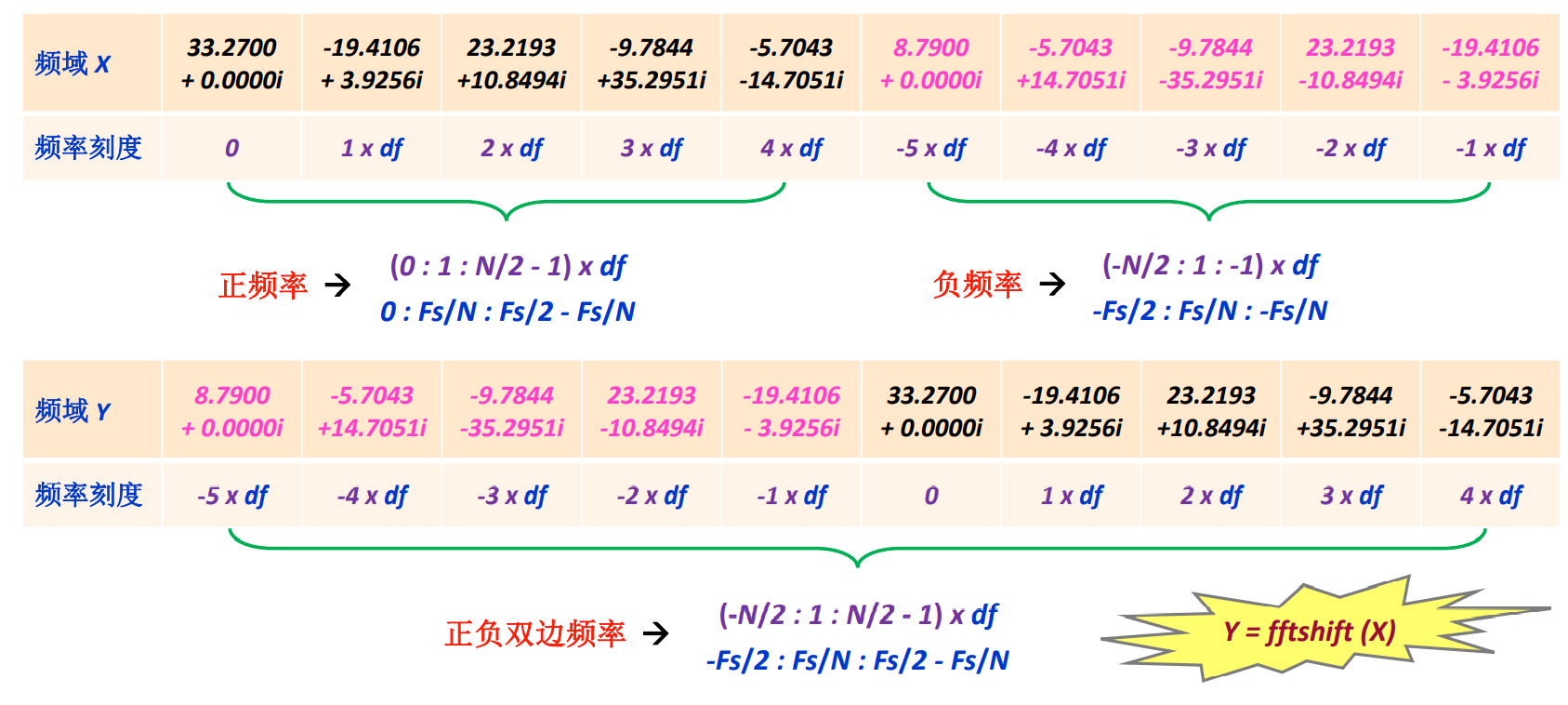# 六 练习 绘制cos信号的频谱图

## 半谱图

clear;clc;close all

%% 定义时域采样信号 x
Fs=100;             % 信号采样频率
Ts=1/Fs;            % 采样时间间隔
N=200;             % 采样信号的长度
t=(0:1:N-1)*Ts;     % 定义信号采样的时间点 t
t=t';               % 为了方便查看, 将行向量 t 转置成列向量

f1=16;              % 第一个余弦信号的频率
f2=45;              % 第二个余弦信号的频率
x=4.5+2.7*cos(2*pi*f1*t+pi/4)+8.2*cos(2*pi*f2*t-pi/6);  % 定义时域采样信号 x

%% 对时域采样信号, 执行快速傅里叶变换 FFT
X=fft(x);           % 执行 FFT 计算, 结果保存在 X 里

%% 提取 X 里正频率的部分, 并且将 X 里负频率的部分合并到正频率
Y=X(1:N/2+1);              % 提取 X 里正频率的部分
Y(2:end-1)=2*Y(2:end-1);   % 将 X 里负频率的部分合并到正频率

%% 计算频域序列 Y 的幅值和相角
A=abs(Y);           % 计算频域序列 Y 的幅值
Pha=angle(Y);       % 计算频域序列 Y 的相角 (弧度制)
R = real(Y);	% 计算频域序列 Y 的实部
I = imag(Y);	% 计算频域序列 Y 的虚部

%% 定义序列 Y 对应的频率刻度
df=Fs/N;            % 频率间隔
f=(0:1:N/2)*df;     % 频率刻度
f=f';               % 为了方便查看, 将行向量 f 转置成列向量

%% 绘制时域采样信号 x 的波形
figure
plot(t,x)
xlabel('时间 [s]')
ylabel('信号值 x(t)')

%% 绘制频域序列 Y 的幅频图 & 相频图
figure
subplot(2,1,1)
plot(f,A)         % 绘制频域序列 Y 的幅频图
grid on
xlabel('频率 [Hz]')
ylabel('Y 的幅值')

subplot(2,1,2)
plot(f,Pha)       % 绘制频域序列 Y 的相频图
grid on
xlabel('频率 [Hz]')
ylabel('Y 的相角')

%% 绘制频域序列 Y 的实部图 & 虚部图
figure
subplot(2,1,1)
plot(f,R)         % 绘制频域序列 Y 的实部图
grid on
xlabel('频率 [Hz]')
ylabel('Y 的实部')

subplot(2,1,2)
plot(f,I)       % 绘制频域序列 Y 的虚部图
grid on
xlabel('频率 [Hz]')
ylabel('Y 的虚部')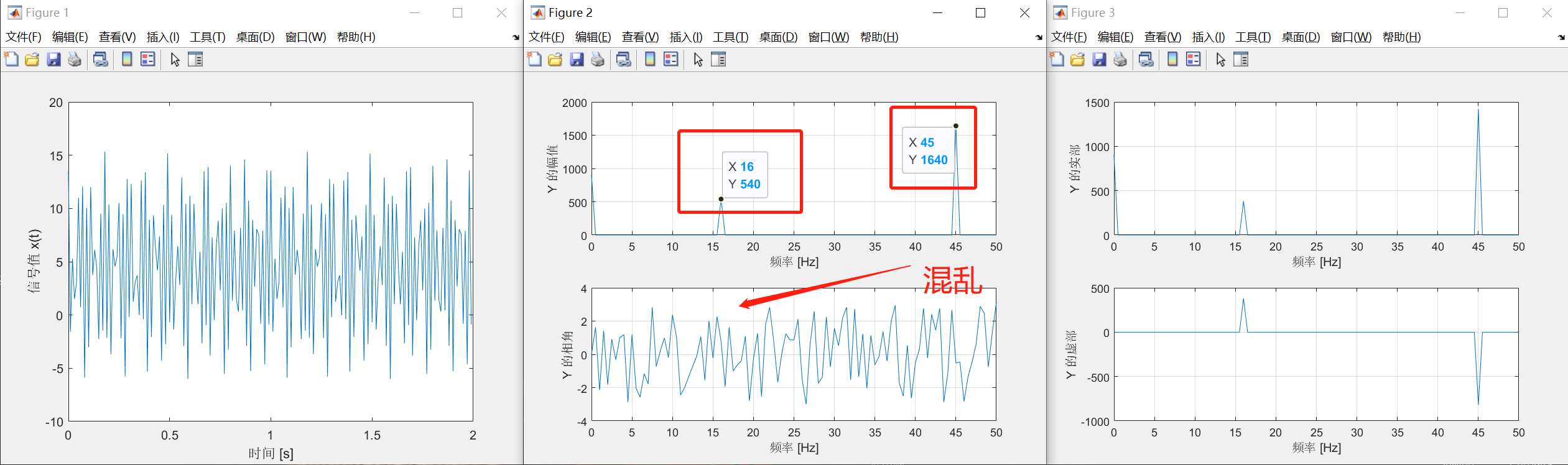%% 消除相位混乱
X(abs(X)<1e-8)=0;   % 将频域序列 X 中, 幅值小于 1e-8 的数值置零

%% 修正频域序列的幅值, 使得 FFT 变换的结果有明确的物理意义
X=X/N;              % 将频域序列 X 除以序列的长度 N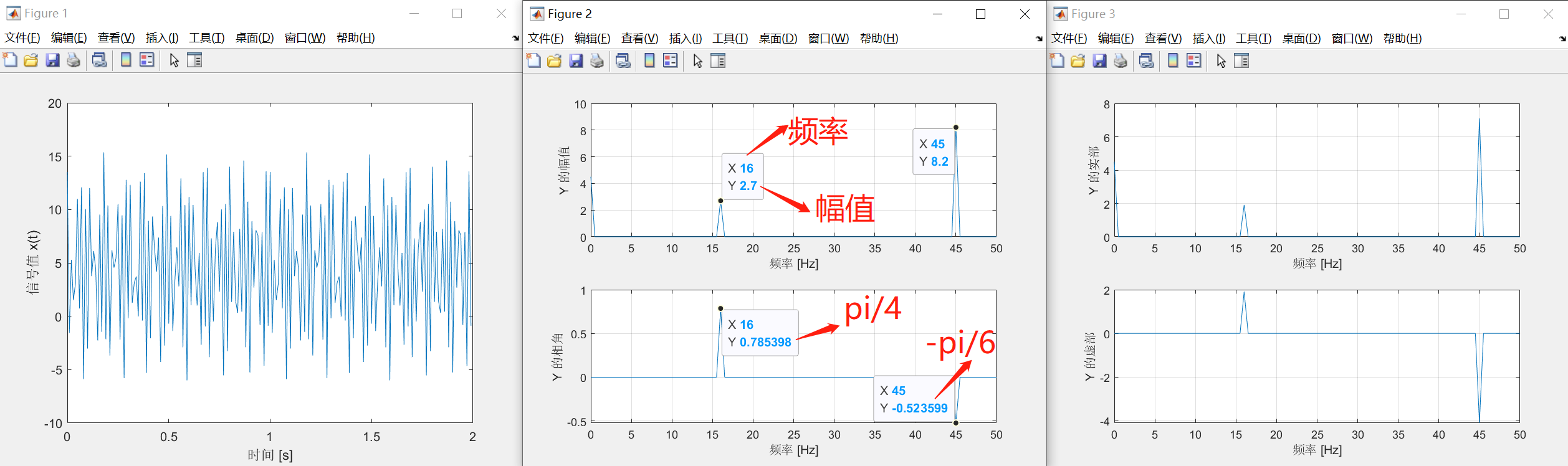x=4.5+2.7cos(2pif1t+pi/4)+8.2cos(2pif2t-pi/6);

## 全谱图


%% 定义时域采样信号 x
Fs=100;             % 信号采样频率
Ts=1/Fs;            % 采样时间间隔
N=200;             % 采样信号的长度
t=(0:1:N-1)*Ts;     % 定义信号采样的时间点 t
t=t';               % 为了方便查看, 将行向量 t 转置成列向量

f1=16;              % 第一个余弦信号的频率
f2=45;              % 第二个余弦信号的频率
x=4.5+2.7*cos(2*pi*f1*t+pi/4)+8.2*cos(2*pi*f2*t-pi/6);  % 定义时域采样信号 x

%% 对时域采样信号, 执行快速傅里叶变换 FFT
X=fft(x);           % 执行 FFT 计算, 结果保存在 X 里

%% 消除相位混乱
X(abs(X)<1e-8)=0;   % 将频域序列 X 中, 幅值小于 1e-8 的数值置零

%% 修正频域序列的幅值, 使得 FFT 变换的结果有明确的物理意义
X=X/N;              % 将频域序列 X 除以序列的长度 N

%% 将 X 重新排列, 把负频率部分搬移到序列的左边, 把正频率部分搬移到序列的右边
Y=fftshift(X);      % 新的频域序列 Y

%% 计算频域序列 Y 的幅值和相角
A=abs(Y);           % 计算频域序列 Y 的幅值
Pha=angle(Y);       % 计算频域序列 Y 的相角 (弧度制)
R = real(Y);	% 计算频域序列 Y 的实部
I = imag(Y);	% 计算频域序列 Y 的虚部

%% 定义序列 Y 对应的频率刻度
df=Fs/N;            % 频率间隔
f=(-N/2:1:N/2-1)*df;     % 频率刻度
f=f';               % 为了方便查看, 将行向量 f 转置成列向量

%% 绘制时域采样信号 x 的波形
figure
plot(t,x)
xlabel('时间 [s]')
ylabel('信号值 x(t)')

%% 绘制频域序列 Y 的幅频图 & 相频图
figure
subplot(2,1,1)
plot(f,A)         % 绘制频域序列 Y 的幅频图
grid on
xlabel('频率 [Hz]')
ylabel('Y 的幅值')

subplot(2,1,2)
plot(f,Pha)       % 绘制频域序列 Y 的相频图
grid on
xlabel('频率 [Hz]')
ylabel('Y 的相角')

%% 绘制频域序列 Y 的实部图 & 虚部图
figure
subplot(2,1,1)
plot(f,R)         % 绘制频域序列 Y 的实部图
grid on
xlabel('频率 [Hz]')
ylabel('Y 的实部')

subplot(2,1,2)
plot(f,I)       % 绘制频域序列 Y 的虚部图
grid on
xlabel('频率 [Hz]')
ylabel('Y 的虚部')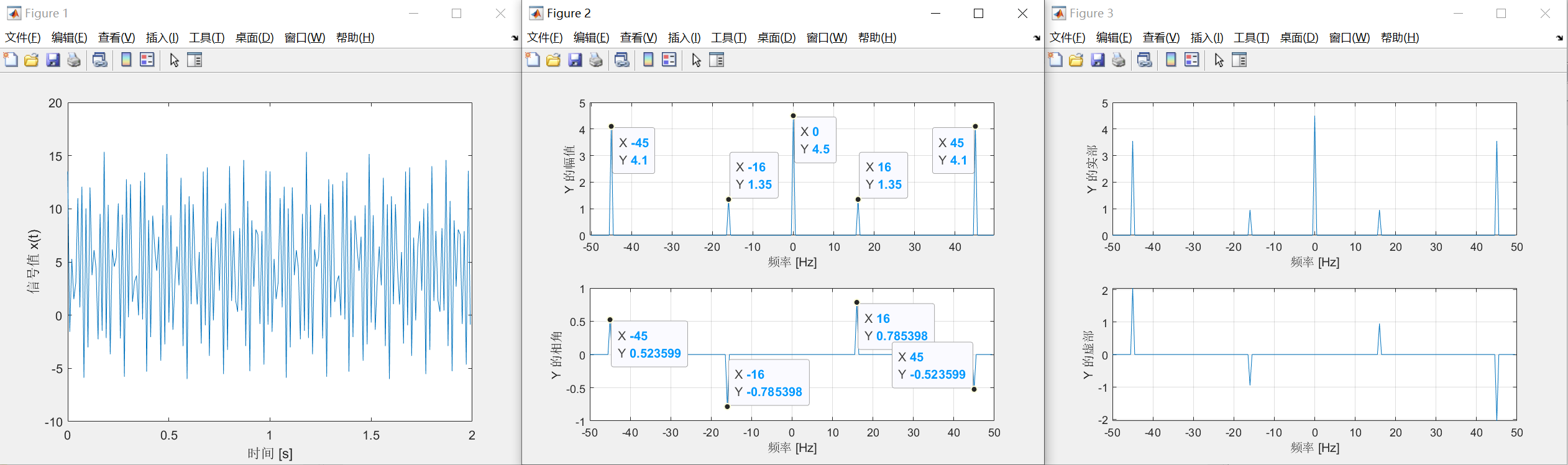# 七，IFFT反傅里叶变换

IX = ifft(X);
%% 绘制频域信号 x 的反傅里叶变换波形
figure
plot(t,IX)
xlabel('时间 [s]')
ylabel('信号值 x(t)')

# 八，采样规则

➢ 时域里采集 N = 100 个数据点 → 频率刻度间隔 df = Fs/N = 20 Hz > (72 – 60 = 12 Hz)

➢ 时域里采集 N = 500 个数据点 → 频率刻度间隔 df = Fs/N = 4 Hz < (72 – 60 = 12 Hz)